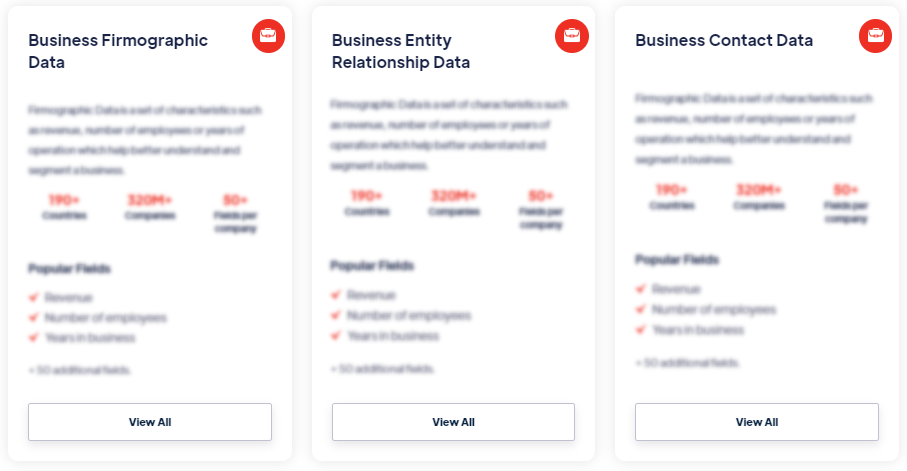# Products

A confusion matrix, also known as an error matrix, is a tabular representation of the performance of a classification model. It summarizes the predictions made by the model on a test dataset and compares them to the actual labels or ground truth values. Read more

#### Our Data Integrations### Confusion Matrix### Browse the Data Marketplace1. What is a confusion matrix?
A confusion matrix, also known as an error matrix, is a tabular representation of the performance of a classification model. It summarizes the predictions made by the model on a test dataset and compares them to the actual labels or ground truth values.

2. What are the components of a confusion matrix?
A confusion matrix consists of four components: true positives (TP), true negatives (TN), false positives (FP), and false negatives (FN). These components represent the number of correctly classified positive instances, correctly classified negative instances, instances that are falsely classified as positive, and instances that are falsely classified as negative, respectively.

3. How is a confusion matrix used?
A confusion matrix provides valuable insights into the performance of a classification model. It allows the calculation of various performance metrics such as accuracy, precision, recall, and F1 score. It helps identify the types of errors made by the model, such as false positives and false negatives, and assesses the model's ability to correctly classify different classes.

4. What metrics can be derived from a confusion matrix?
Several performance metrics can be calculated using a confusion matrix, including accuracy, precision, recall (sensitivity), specificity, F1 score, and the area under the receiver operating characteristic (ROC) curve. These metrics provide different aspects of the model's performance, such as overall correctness, ability to predict positive instances, ability to predict negative instances, and the trade-off between precision and recall.

5. How is a confusion matrix interpreted?
The interpretation of a confusion matrix depends on the specific problem and the desired outcome. Generally, a higher number of true positives and true negatives indicates better model performance. However, the interpretation may vary depending on the relative importance of false positives and false negatives in the specific context. For example, in medical diagnosis, false negatives (missing actual positive cases) may be more critical than false positives.

6. Can a confusion matrix handle multi-class classification?
Yes, a confusion matrix can be extended to handle multi-class classification problems. In this case, the matrix is expanded to include cells representing each class's true positives, true negatives, false positives, and false negatives. The performance metrics derived from the confusion matrix, such as precision and recall, can be calculated for each class individually or summarized using macro- or micro-averaging techniques.

7. What are the limitations of a confusion matrix?
While a confusion matrix provides valuable insights into the performance of a classification model, it has some limitations. It assumes a fixed threshold for classification, which may not be optimal for all scenarios. Additionally, it does not capture the uncertainty associated with predicted probabilities. Further evaluation measures like precision-recall curves or ROC curves may be necessary to fully assess a model's performance.